﻿ Agitation of liquid by a cylindrical mixer - XSim

# Agitation of liquid by a cylindrical mixer

Update: July 1, 2017
OpenFOAM 4.x

## Case directory

\$FOAM_TUTORIALS/multiphase/interFoam/laminar/mixerVessel2D

## Summary

We analyze a mixer as shown in the figure below using MRF function.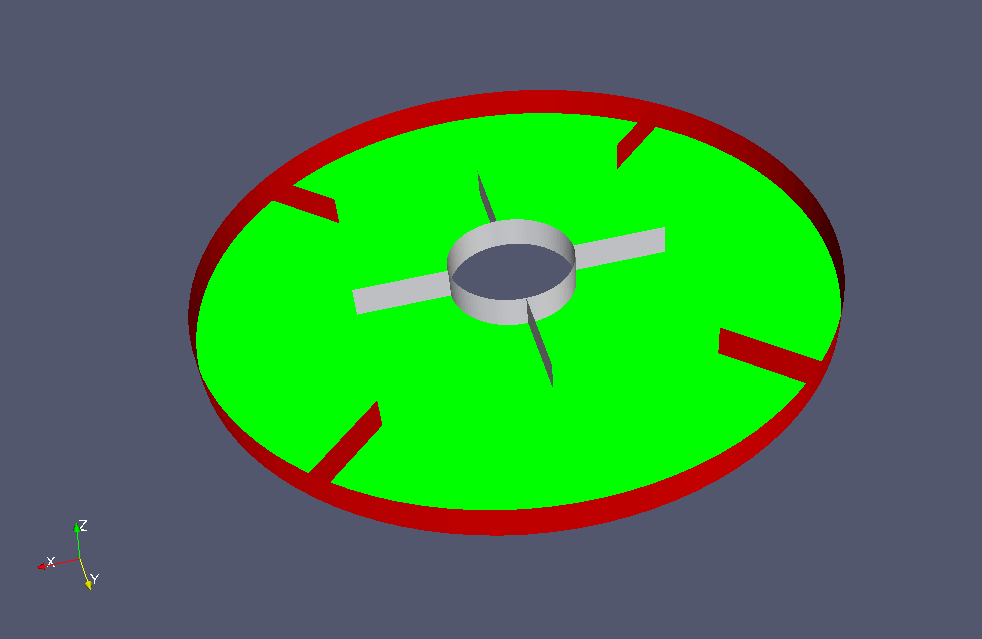Model geometry

The region "rotor" rotates at 6.2831853 rad/s with the Z-axis as the axis of rotation, and agitate the water that occupies 1/4 of the mixer at initial time. We set the gravity acceleration to (0, 0, 0) and do not consider gravity. The calculation is performed as a two-dimensional problem with a single mesh in the Z direction.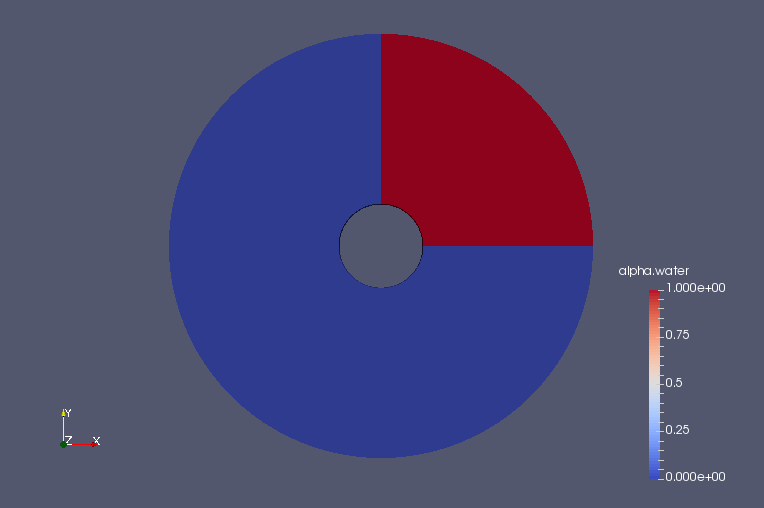Volume ratio of water at initial time (alpha.water)

The rotation axis and the rotation speed are defined in the file constant/MRFProperties as follows.

```MRF1
{
cellZone    rotor;
active      yes;

// Fixed patches (by default they 'move' with the MRF zone)
nonRotatingPatches ();

origin    (0 0 0);
axis      (0 0 1);
omega     constant 6.2831853;
}
```

The rotation area is defined as the cell zone "rotor" as shown in the figure below (white part).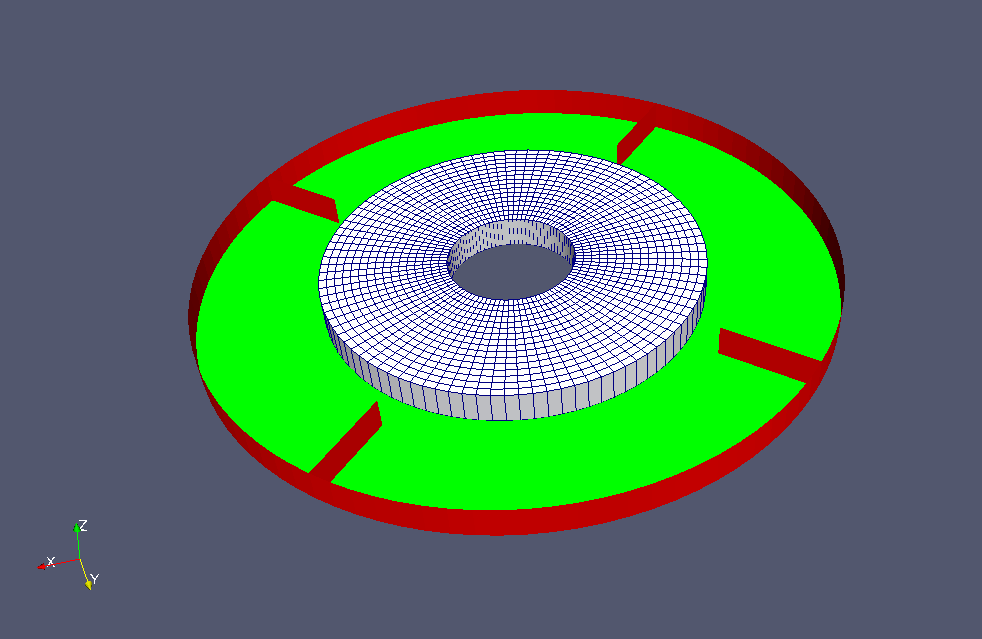Cell zone "rotor"

The meshes are as follows, and the number of mesh is 3072.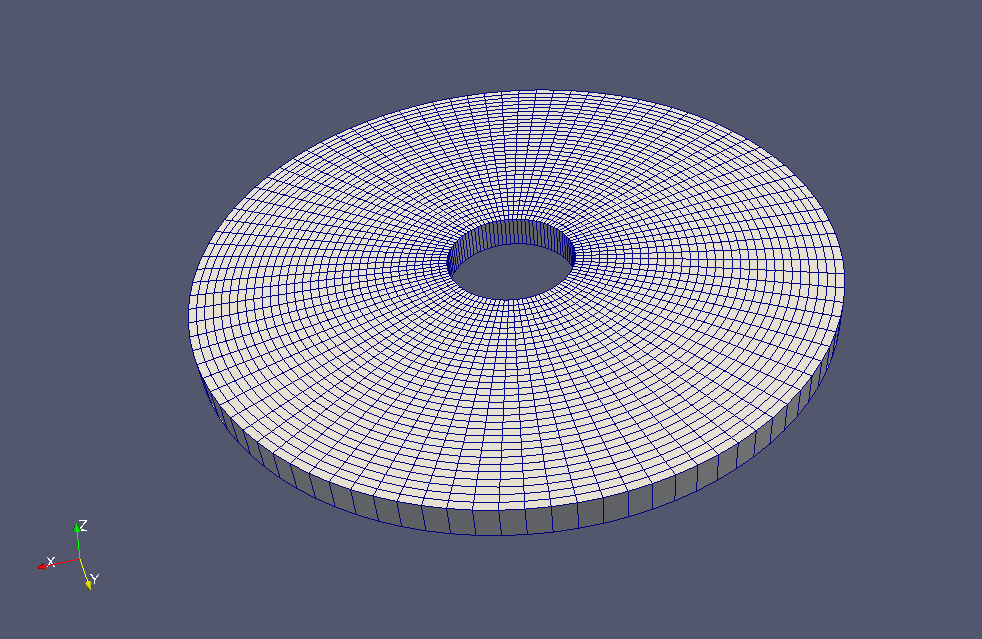Meshes

The calculation result is as follows.

Volume ratio of water (alpha.water)Volume ratio of water at initial time (alpha.water)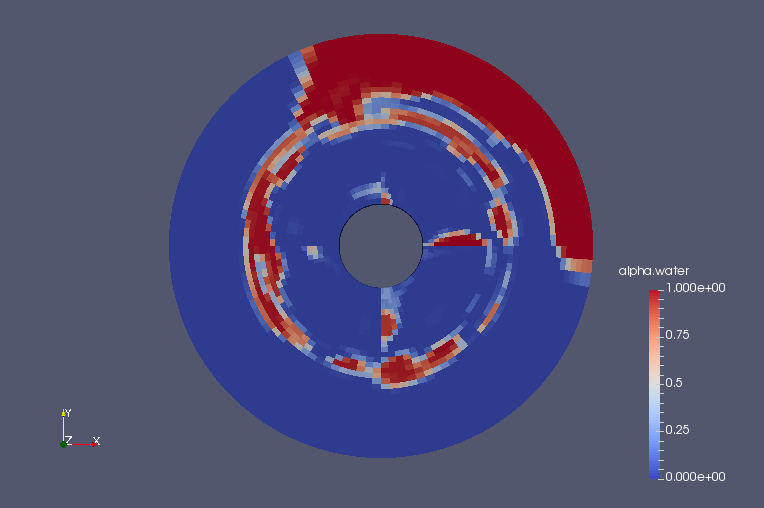Volume ratio of water at final time (alpha.water)

## Commands

cp -r \$FOAM_TUTORIALS/multiphase/interFoam/laminar/mixerVessel2D mixerVessel2D
cd mixerVessel2D

m4 < system/blockMeshDict.m4 > system/blockMeshDict
blockMesh
topoSet
setsToZones -noFlipMap

cp 0/alpha.water.orig 0/alpha.water
setFields
interFoam

paraFoam

## Calculation time

18.25 seconds *Single, Inter(R) Core(TM) i7-2600 CPU @ 3.40GHz 3.40GHz